Electricity & Megnetism

# Ampere’s Circuital law

## What is ampere’s law?

According to Ampere’s law “The line integral of magnetic field B along a closed path due to current is equal to the product of the permeability of free space and the current enclosed by the closed path”.

Mathematically it is expressed as: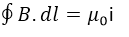Where

μ0=permeability of free space

i=current flowing through the conductor.

Proof: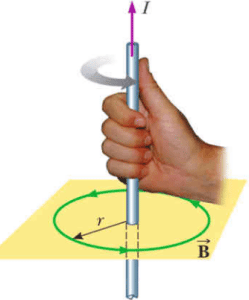Consider a straight conductor in which current i is flowing. The current produces the magnetic field B around the conductor. The magnetic field lines are in the form of concentric circles.

Ampere showed that the flux density B at any point near the conductor is directly proportional to the current i and inversely proportional to the distance ‘r’ from the conductor,so: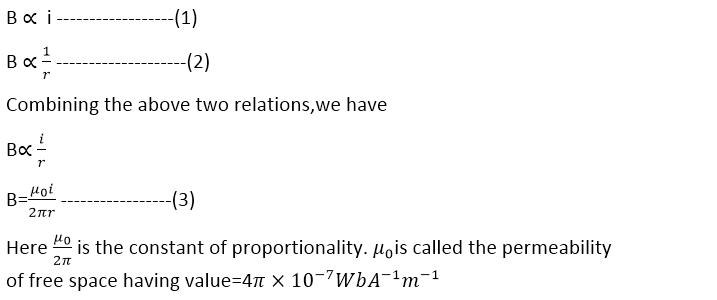Where is the length of the path called the circumference of the circle?

Divide the circle representing the magnetic field line into a large number of small elements each of length dl. The quantity B.dl is calculated for each element as:

B.dl=Bdlcos= Bdlcos0=Bdl

For complete circle: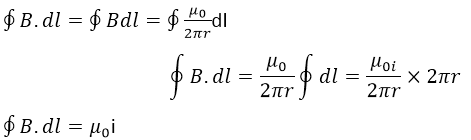## Integral form of ampere’s  circuital law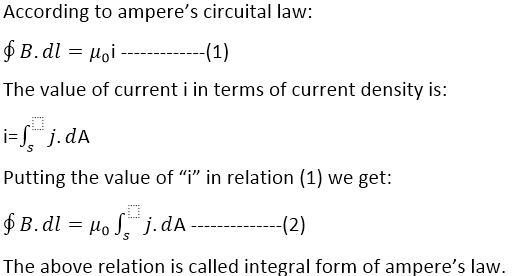## Differential form of ampere law

Since the integral form of ampere’s law is: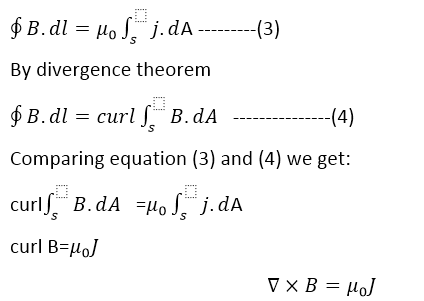The above relation is known as a differential form of ampere’s circuital law.

## Applications of ampere’s circuital law

• ### Field due to a solenoid:

Consider a solenoid having n turns per unit length. When current passes, through the solenoid, the magnetic field is produced inside the solenoid which is directed along the axis of the solenoid. The magnetic field in space outside is so weak that it is considered as zero.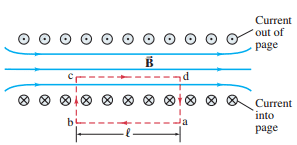In order to calculate the value of magnetic field B inside the solenoid using ampere’s law, we consider a closed path abcda in the form of a rectangle. This closed path is known as the Amperian path, as seen in the figure.
Let this path be divided into four elements of lengths as:

ab =L1

bc = L2

cd=L3

da=L4

Such that the sum of dot product of magnetic field and length of the element is:

∑ B.ΔL =BL1 Cos θ1 + BL2 Cos θ2+BL3 Cos θ3+BL4 Cos θ4   ……….(1)

As L1 is parallel to the magnetic field lines inside the solenoid, therefore, θ=0°

BL1 Cos θ1=BL1

L2 and L4 are perpendicular to the magnetic field i.e θ2=90° and θ4=90°

BL2 Cos θ2=0

BL4 Cos θ4=0

and L3 lines outside the solenoid where the field is weaker,i.e B=0

oR     BL3 Cos θ3=0

Put all these values in equation (1) we get:

∑ B.ΔL=BL1+0+0+0

∑ B.ΔL=BL1       …………..(2)

According to the Ampere’s law

∑ B.ΔL=μ0I          ………(3)

If N is the number of turns of coil then

Current =NI

And if “n” is the number of turns per unit length,then

n = N/L1

N=nL1

Current = n L1I

∑ B.ΔL =μ0n L1I     …………(4)

By comparing equation (2) and (4) ,we get

μ0n L1I=BL1

B =μ0nI

Related topics in our website are: